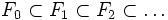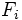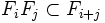Filtered ring

(diff) ← Older revision | Latest revision (diff) | Newer revision → (diff)

Definition

A filtered ring is a commutative unital ring$A$ equipped with a filtration, viz., a structure of an ascending chain of subgroups:$F_0 \subset F_1 \subset F_2 \subset \ldots$

such that the following hold:

• The union of the$F_i$s is$A$
• Each$F_i$ is a subgroup under addition
•$1 \in F_0$
•$F_iF_j \subset F_{i+j}$

It turns out from these that$F_0$ is a unital subring.

Related notions

• Graded ring: Any graded ring naturally becomes a filtered ring. The filtration associated with the gradation is the filtration where$F_i$ is the sum of the graded pieces from$0$ to$i$.